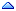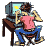Home » Other » General » Puzzle n°10 - All possible solution for Fibonacci Series *
Puzzle n°10 - All possible solution for Fibonacci Series * Sat, 18 October 2008 04:03rajavu1 Messages: 1574Registered: May 2005 Location: Bangalore , India Senior MemberLet me invite all the possible solution for generating Fibonacci Series in SQL or PL/SQL.

 Quote: In mathematics, the Fibonacci numbers are a sequence of numbers named after Leonardo of Pisa, known as Fibonacci.The first number of the sequence is 0, the second number is 1, and each subsequent number is equal to the sum of the previous two numbers of the sequence itself, yielding the sequence 0, 1, 1, 2, 3, 5, 8, etc.

The output should be as follows .

Code:
```             0
1
1
2
3
5
8
13
21
34
55
89```Rajuvan.

[Updated on: Sun, 19 October 2008 08:00]

Report message to a moderator

Re: Puzzle n°10 - All possible solution for Fibonacci Series [message #354407 is a reply to message #354373] Sat, 18 October 2008 09:17Michel Cadot Messages: 68453Registered: March 2007 Location: Nanterre, France, http://... Senior MemberAccount Moderator
Here are 2 solutions (I choosed the easiest way):
```SQL> DEFINE N=12
SQL> SELECT ' F('||num||') = '||fibo "Fibonacci"
2  FROM ( SELECT 1 rn FROM DUAL )
3  MODEL
4    DIMENSION BY (rn)
5    MEASURES (0 num, 1 fibo)
6    RULES
7      ITERATE (&N)
8      ( num[ITERATION_NUMBER] = ITERATION_NUMBER,
9        fibo[ITERATION_NUMBER] =
10          CASE
11          WHEN ITERATION_NUMBER IN (0,1) THEN ITERATION_NUMBER
12          ELSE fibo[CV()-1] + fibo[CV()-2]
13          END
14      )
15  ORDER BY num
16  /
Fibonacci
------------------------------------------------------------------
F(0) = 0
F(1) = 1
F(2) = 1
F(3) = 2
F(4) = 3
F(5) = 5
F(6) = 8
F(7) = 13
F(8) = 21
F(9) = 34
F(10) = 55
F(11) = 89

12 rows selected.

SQL> SELECT ' F('||rn||') = '||fibo "Fibonacci"
2  FROM ( SELECT rownum-1 rn FROM DUAL CONNECT BY LEVEL <= &N )
3  MODEL
4    DIMENSION BY (rn)
5    MEASURES (1 fibo)
6    RULES
7      ( fibo[ANY] =
8          CASE
9          WHEN CV(rn) IN (0,1) THEN CV(rn)
10          ELSE fibo[CV()-1] + fibo[CV()-2]
11          END
12      )
13  ORDER BY rn
14  /
Fibonacci
------------------------------------------------------------------
F(0) = 0
F(1) = 1
F(2) = 1
F(3) = 2
F(4) = 3
F(5) = 5
F(6) = 8
F(7) = 13
F(8) = 21
F(9) = 34
F(10) = 55
F(11) = 89

12 rows selected.```

Regards
Michel

[Updated on: Sun, 19 October 2008 00:23]

Report message to a moderator

Re: Puzzle n°10 - All possible solution for Fibonacci Series [message #354421 is a reply to message #354373] Sat, 18 October 2008 14:59ebrian Messages: 2794Registered: April 2006 Senior Member
```SQL> select level - 1 "LEVEL"
2     , (1 / sqrt(5)) *
3     (power(((1 + sqrt(5))/2), level - 1) - power(((1 - sqrt(5))/2), level - 1)) fibonacci
4  from dual
5  connect by level < 13;

LEVEL  FIBONACCI
---------- ----------
0          0
1          1
2          1
3          2
4          3
5          5
6          8
7         13
8         21
9         34
10         55
11         89

12 rows selected.
```

[Updated on: Sat, 18 October 2008 15:04]

Report message to a moderator

Re: Puzzle n°10 - All possible solution for Fibonacci Series [message #354441 is a reply to message #354421] Sun, 19 October 2008 00:27Michel Cadot Messages: 68453Registered: March 2007 Location: Nanterre, France, http://... Senior MemberAccount Moderator
Binet's formula. Neat.Regards
Michel

[Updated on: Sun, 19 October 2008 00:30]

Report message to a moderator

Re: Puzzle n°10 - All possible solution for Fibonacci Series [message #354463 is a reply to message #354373] Sun, 19 October 2008 07:59rajavu1 Messages: 1574Registered: May 2005 Location: Bangalore , India Senior MemberGood ..

Anyway my favorite method is Table function . PL/SQL way of doing it.

```SQL> CREATE OR REPLACE TYPE FIBONACCI as table OF NUMBER(10);
2  /

Type created.

SQL> CREATE OR REPLACE FUNCTION gen_fibonacci (series_length IN NUMBER)
2  RETURN FIBONACCI PIPELINED
3  AS
4  n0 NUMBER :=0;
5  n1 NUMBER :=1;
6  n2 NUMBER :=0;
7  BEGIN
8      PIPE ROW(n0);
9      PIPE ROW(n1);
10    FOR i IN n0..series_length-3
11    LOOP
12      n2 := n0 + n1 ;
13      PIPE ROW(n2);
14      n0 := n1;
15      n1 := n2;
16    END LOOP;
17    RETURN;
18  END gen_fibonacci;
19  /

Function created.

SQL> select COLUMN_VALUE FIBONACCI
2  from TABLE(gen_fibonacci(10));

FIBONACCI
----------
0
1
1
2
3
5
8
13
21
34

10 rows selected.

SQL>```Rajuvan.
Re: Puzzle n°10 - All possible solution for Fibonacci Series [message #354477 is a reply to message #354441] Sun, 19 October 2008 10:39ebrian Messages: 2794Registered: April 2006 Senior Member
 Michel Cadot wrote on Sun, 19 October 2008 01:27 Binet's formula. Neat.Yeah, but the MODEL looks cooler !!

Rajuvan...nice use of PIPELINED.
Re: Puzzle n°10 - All possible solution for Fibonacci Series * [message #539497 is a reply to message #354373] Mon, 16 January 2012 10:11Barbara Boehmer Messages: 9005Registered: November 2002 Location: California, USA Senior Member
rajavu1 wrote on Sat, 18 October 2008 02:03

Let me invite all the possible solution for generating Fibonacci Series in SQL or PL/SQL.

Here is an 11g recursive sub-query factoring method that was not available yet when this thread was started.

```SCOTT@orcl_11gR2> with
2    numbers (lvl, fibonacci, next) as
3  	 (select 0, 0, 1
4  	  from	 dual
5  	  union  all
6  	  select lvl + 1, next, fibonacci + next
7  	  from	 numbers
8  	  where  lvl <= 10)
9  select lvl "LEVEL", fibonacci
10  from   numbers
11  order  by lvl
12  /

LEVEL  FIBONACCI
---------- ----------
0          0
1          1
2          1
3          2
4          3
5          5
6          8
7         13
8         21
9         34
10         55
11         89

12 rows selected.
```

Re: Puzzle n°10 - All possible solution for Fibonacci Series * [message #539512 is a reply to message #539497] Mon, 16 January 2012 11:20Michel Cadot Messages: 68453Registered: March 2007 Location: Nanterre, France, http://... Senior MemberAccount Moderator
Yeap! When I saw you answered on this topic I knew you had added this solution before reading it.Regards
Michel

[Updated on: Mon, 16 January 2012 11:20]

Report message to a moderator

 Previous Topic: 32bit VS 64bit Next Topic: How to recover deleted files
Goto Forum:

Current Time: Sat Jun 03 00:45:00 CDT 2023